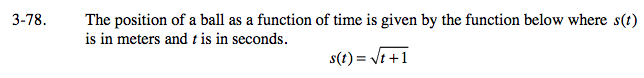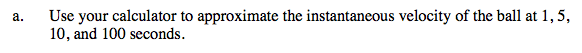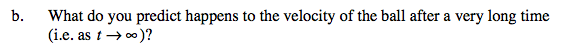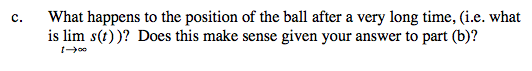### Home > CALC > Chapter Ch3 > Lesson 3.2.3 > Problem3-78

3-78.Instantaneous velocity = Instantaneous Rate of Change (IROC) = Derivative

$\text{Rewrite }s(t)\text{ using exponents: }s(t)=(t+1)^{1/2}$

Find s'(t) using the Power rule.

Evaluate s'(1), s'(5), s'(10) and s'(100).Note: t = 100 seconds is a very long time for a ball to be in motion. (This must be an unearthly situation!) Is s(t) increasing, decreasing or neither as t→∞? Is s(t) changing rapidly or not so rapidly? Explain.Does a square root function (such as s(t)) have a horizontal asymptote as t→∞?